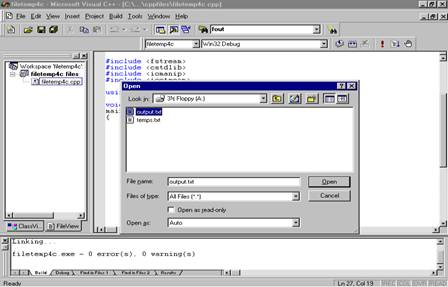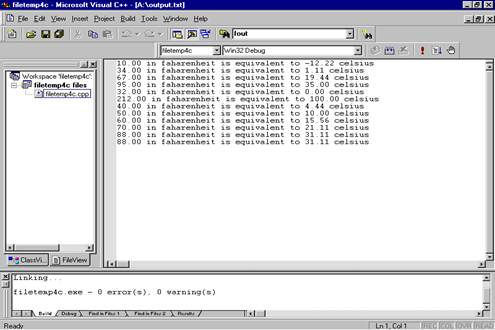FILE INPUT/OUTPUT

Let’s say we wish to find the average of 100 temperatures.  We design a C++ program containing a while loop whose execution is regulated with a sentinel.  What would happen if we typed in the 75th temperature incorrectly?

A file is a collection of related data items stored on an external storage device such as a hard disk.

A text file is a sequence of ASCII coded characters.

Input and output using text files in a C++ program is done using the C++ stream class.  The data type of the stream object for input is ifstream, and the data type of the stream object for output is ofstream.  These stream variables are in the fstream header file.

To use a file for input we need to have the operating system open the file.  When we open a file, the operating system designates whether the file is to be for input (is read from) or output (written to) and then sets a file pointer to the beginning of the file.  The file pointer keeps track of what portion of the file has been processed and which portion hasn’t.

The open member function of a stream variable opens the file as either input or output depending on whether the stream variable is an input or output stream variable.  The file pointer is positioned at the beginning of the file.  If the file didn’t previously exist then the operating system creates one.  If it did exist, then its previous contents are erased and overwritten,

The extraction operator  >>  is used in the same manner with the file input as cin is used for standard input.  The data is extracted from the file and placed into the variable’s memory location.

At the end of a program we must close the file.  The close member function is used.  The closed member function sends any data in the stream to the external file, saves the external file, and detaches the file from ofstream so that no more output is allowed.

Assume we create a data file called temps.txt that contains the following data:

10

34

67

95

32

212

40

50

60

70

88

#include <fstream>

#include <iomanip>

#include <iostream>

using namespace std;

int

main()

{

float temp, totaltemp = 0, averagetemp;

int num_temps, count = 0;

ifstream tempin;  //declare an input stream variable

tempin.open("temps.txt"); //open the file temps.txt for input

tempin >> num_temps;  //read from temps.txt

cout << "The number of records is: " << num_temps << endl;

cout << fixed << showpoint << setprecision(2);

while(count < num_temps)

{

tempin >> temp;

cout << temp << " in Fahrenheit is equivalent to " << 5.0/9 * (temp - 32)

<< " Celsius" << endl;

totaltemp += temp;

count++;

}

cout << "The average temperature is: " << totaltemp/count << " Fahrenheit." << endl;

tempin.close();  //close the input file

return 0;

}

The number of records is: 10

34.00 in Fahrenheit is equivalent to 1.11 Celsius

67.00 in Fahrenheit is equivalent to 19.44 Celsius

95.00 in Fahrenheit is equivalent to 35.00 Celsius

32.00 in Fahrenheit is equivalent to 0.00 Celsius

212.00 in Fahrenheit is equivalent to 100.00 Celsius

40.00 in Fahrenheit is equivalent to 4.44 Celsius

50.00 in Fahrenheit is equivalent to 10.00 Celsius

60.00 in Fahrenheit is equivalent to 15.56 Celsius

70.00 in Fahrenheit is equivalent to 21.11 Celsius

88.00 in Fahrenheit is equivalent to 31.11 Celsius

The average temperature is: 74.80 Fahrenhet.

Press any key to continue

#include <fstream>

#include <iomanip>

#include <iostream>

using namespace std;

int

main()

{

float temp, totaltemp = 0;

int count = 0;

ifstream tempin;

tempin.open("temps.txt");

cout << fixed << showpoint << setprecision(2);

while(!tempin.eof())

{

tempin >> temp;

cout << temp << " in Fahrenheit is equivalent to " << 5.0/9 * (temp - 32)

<< " Celsius" << endl;

totaltemp += temp;

count++;

}

cout << "The average temperature is: " << totaltemp/count << " Fahrenheit." << endl;

tempin.close();

return 0;

}

10.00 in Fahrenheit is equivalent to -12.22 Celsius

34.00 in Fahrenheit is equivalent to 1.11 Celsius

67.00 in Fahrenheit is equivalent to 19.44 Celsius

95.00 in Fahrenheit is equivalent to 35.00 Celsius

32.00 in Fahrenheit is equivalent to 0.00 Celsius

212.00 in Fahrenheit is equivalent to 100.00 Celsius

40.00 in Fahrenheit is equivalent to 4.44 Celsius

50.00 in Fahrenheit is equivalent to 10.00 Celsius

60.00 in Fahrenheit is equivalent to 15.56 Celsius

70.00 in Fahrenheit is equivalent to 21.11 Celsius

88.00 in Fahrenheit is equivalent to 31.11 Celsius

The average temperature is: 68.91 Fahrenheit.

Press any key to continue

What do you do when you don’t know the number of data items in your file?  The eof member function allows us to test for the end of the file.   The function returns a true value when the next character of the output stream is the end of file character and a false value otherwise.

#include <fstream>

#include <iomanip>

#include <cstdlib>

#include <iostream>

using namespace std;

int

main()

{

float temp, totaltemp = 0;

int count = 0;

ifstream tempin;

tempin.open("temps.txt");

cout << fixed << showpoint << setprecision(2);

while(!tempin.eof())

{

tempin >> temp;

cout << temp << " in Fahrenheit is equivalent to " << 5.0/9 * (temp - 32)

<< " Celsius" << endl;

totaltemp += temp;

count++;

}

cout << "The average temperature is: " << totaltemp/count << " Fahrenheit." << endl;

tempin.close();

return 0;

}

What happens when we try to open a file that doesn’t exist? Use the fail member function to test for an open failure.
#include <fstream>

#include <iomanip>

#include <cstdlib>

#include <iostream>

using namespace std;

int

main()

{

float temp, totaltemp = 0;

int count = 0;

ifstream tempin;

tempin.open("temps.txt");

if (tempin.fail())  //using fail member function to test for faliure

{

cerr << "File failed to open";

abort();  //program is terminated

}

cout << fixed << showpoint << setprecision(2);

while(!tempin.eof())

{

tempin >> temp;

cout << temp << " in Fahrenheit is equivalent to " << 5.0/9 * (temp - 32)

<< " Celsius" << endl;

totaltemp += temp;

count++;

}

cout << "The average temperature is: " << totaltemp/count << " Fahrenheit." << endl;

tempin.close();

return 0;

}

10.00 in Fahrenheit is equivalent to -12.22 Celsius

34.00 in Fahrenheit is equivalent to 1.11 Celsius

67.00 in Fahrenheit is equivalent to 19.44 Celsius

95.00 in Fahrenheit is equivalent to 35.00 Celsius

32.00 in Fahrenheit is equivalent to 0.00 Celsius

212.00 in Fahrenheit is equivalent to 100.00 Celsius

40.00 in Fahrenheit is equivalent to 4.44 Celsius

50.00 in Fahrenheit is equivalent to 10.00 Celsius

60.00 in Fahrenheit is equivalent to 15.56 Celsius

70.00 in Fahrenheit is equivalent to 21.11 Celsius

88.00 in Fahrenheit is equivalent to 31.11 Celsius

The average temperature is: 68.91 Fahrenheit.

Press any key to continue

We can write to an output file instead of the standard output (monitor) using an ofstream variable and the member functions just discussed.

#include <fstream>

#include <cstdlib>

#include <iomanip>

#include <iostream>

using namespace std;

void

main()

{

float temp;

ifstream tempin;

ofstream tempout;

tempout.open("output.txt");

if (tempout.fail())

{

cerr << "File failed to open:output.txt";

abort();

}

tempin.open("temps.txt");

if (tempin.fail())

{

cerr << "File failed to open: temps.txt";

abort();

}

tempout << fixed << showpoint << setprecision(2);

while(!tempin.eof())

{

tempin >> temp;

tempout << temp << " in Fahrenheit is equivalent to " << 5.0/9 * (temp - 32)

<< " Celsius" << endl;

}

tempin.close();

tempout.close();

}